# Bar Charts in Python using Plotly

## Bar Charts in Python using Plotly

In this article, I am going to discuss Bar Charts in Python using Plotly with Examples. Please read our previous article where we discussed Scatter and Bubble Plots in Python using Plotly with Examples.

##### Bar Charts using Plotly in Python

The height of each bar shows the number of occurrences in that category, and bar charts are most typically used to illustrate categorical data. To plot a Bar Plot in Plotly, just call the bar() function of the Plotly Express (px) instance and pass correct data to the x and y inputs.

###### Example 1 –
```# Import library
import plotly.express as px

# Create a bar plot for categorical data
xd = ['Category 1', 'Category 2', 'Category 3']
yd = [5, 3, 6]

figure = px.bar(x=xd, y=yd)
figure.show()
```
###### Output: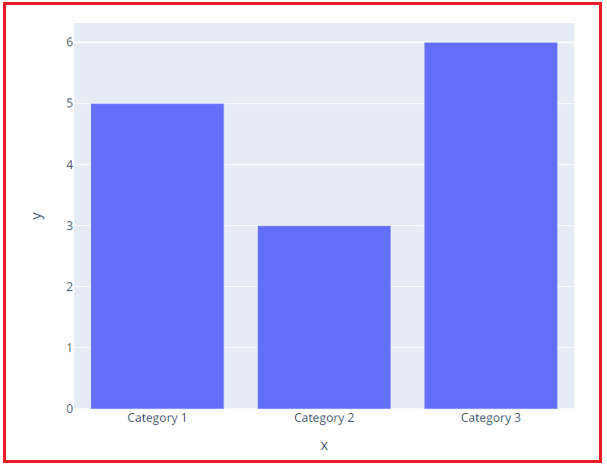###### Example 2 – Creating a bar plot for a dataset
```# Import Library
import plotly.express as px

data = px.data.iris()

# Creating a bar plot for a dataset
figure = px.bar(data, x="sepal_width", y="sepal_length")
figure.show()
```
###### Output: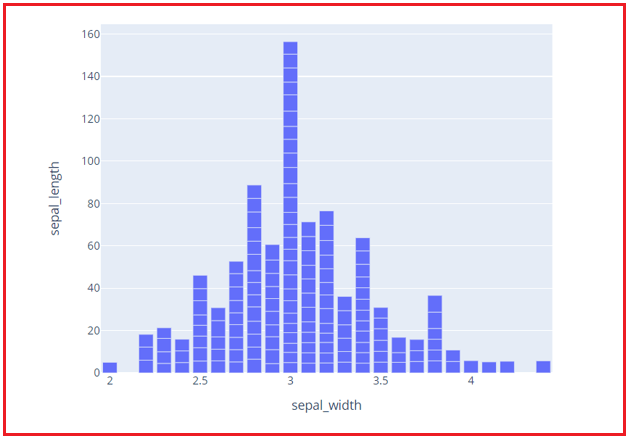###### Example 3 – Customizing a bar plot for a dataset by color
```# Import Library
import plotly.express as px

data = px.data.iris()

# Customizing bar plot according to color
figure = px.bar(data, x="sepal_width", y="sepal_length", color="species")
figure.show()
```
###### Output: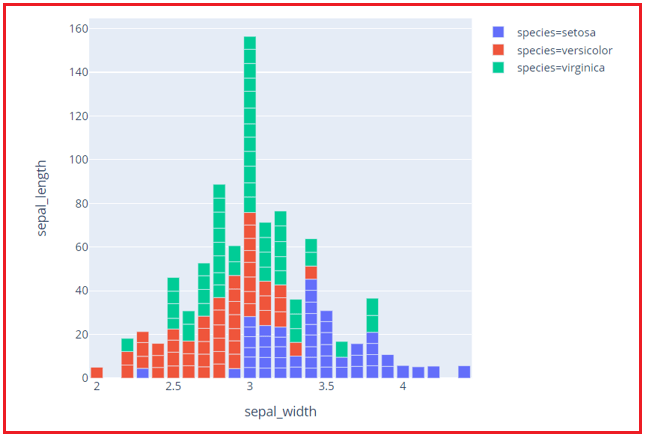###### Example 4 – Creating a horizontal bar plot
```# Import Library
import plotly.express as px

data = px.data.iris()

# Creating a horizontal bar plot
figure = px.bar(data, x="sepal_width", y="sepal_length", color="species", orientation="h")
figure.show()
```
###### Output: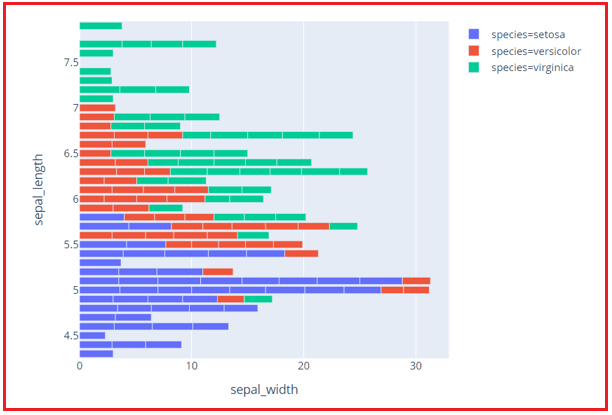###### Example 5 – Creating an ordered bar plot
```# Import library
import plotly.express as px

xd = ['Category 1', 'Category 2', 'Category 3']
yd = [5, 3, 6]

# Create an ordered bar plot for categorical data
figure = px.bar(x=xd, y=yd)
figure.update_layout(xaxis={'categoryorder':'total descending'})
figure.show()
```
###### Output: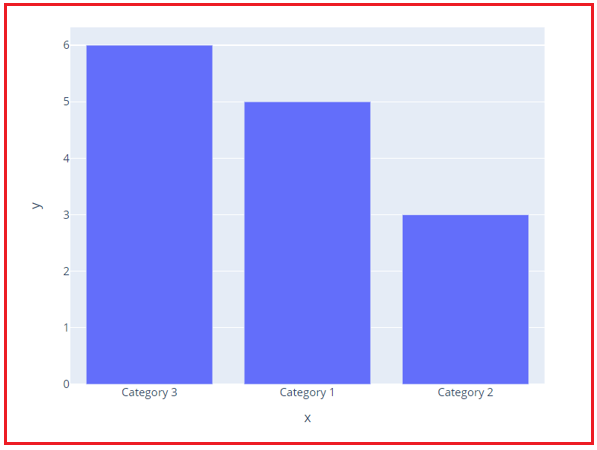###### Example 6 – Creating a grouped bar plot
```# Import Library
import plotly.express as px
data = px.data.iris()

# Customizing bar plot according to color
figure = px.bar(data, x="sepal_width", y="sepal_length", color="species", barmode="group")
figure.show()
```
###### Output: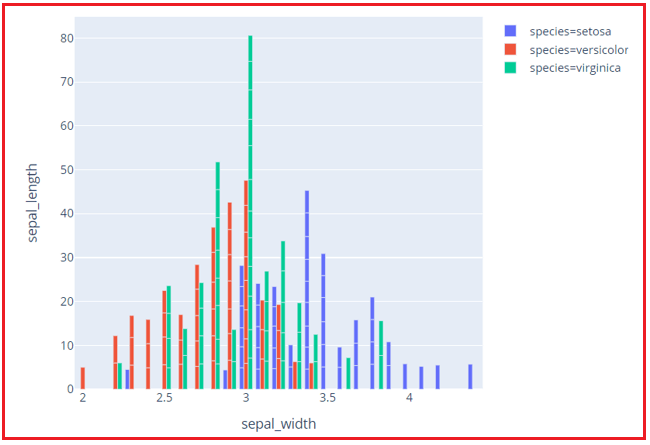In the next article, I am going to discuss Box Plots and Dist Plots in Python using Plotly for Data Science with Examples. Here, in this article, I try to explain Bar Charts in Python using Plotly for Data Science with Examples. I hope you enjoy this Bar Charts in Python using Plotly for Data Science article.